#### Kenward-Roger Degrees of Freedom Approximation

The DDFM=KENWARDROGER option prompts PROC GLIMMIX to compute the denominator degrees of freedom in t tests and F tests by using the approximation described in Kenward and Roger (1997). For inference on the linear combination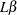in a Gaussian linear model, they propose a scaled Wald statistic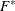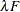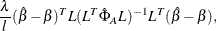where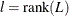,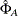is a bias-adjusted estimator of the precision of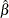, and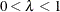. An appropriate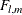approximation to the sampling distribution ofis derived by matching the first two moments ofwith those from the approximating F distribution and solving for the values ofand m. The value of m thus derived is the Kenward-Roger degrees of freedom. The precision estimatoris bias-adjusted, in contrast to the conventional precision estimator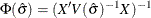, which is obtained by simply replacingwith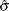in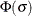, the asymptotic variance of. This method usesto address the fact that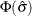is a biased estimator of, anditself underestimates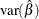whenis unknown. This bias-adjusted precision estimator is also discussed in Prasad and Rao (1990); Harville and Jeske (1992); Kackar and Harville (1984).

By default, the observed information matrix of the covariance parameter estimates is used in the calculations. For covariance structures that have nonzero second derivatives with respect to the covariance parameters, the Kenward-Roger covariance matrix adjustment includes a second-order term. This term can result in standard error shrinkage. Also, the resulting adjusted covariance matrix can then be indefinite and is not invariant under reparameterization. The FIRSTORDER suboption of the DDFM=KENWARDROGER option eliminates the second derivatives from the calculation of the covariance matrix adjustment. For scalar estimable functions, the resulting estimator is referred to as the Prasad-Rao estimator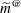in Harville and Jeske (1992). You can use the COVB(DETAILS) option to diagnose the adjustments that PROC GLIMMIX makes to the covariance matrix of fixed-effects parameter estimates. An application with DDFM=KENWARDROGER is presented in Example 41.8. The following are examples of covariance structures that generally lead to nonzero second derivatives: TYPE=ANTE(1), TYPE=AR(1), TYPE=ARH(1), TYPE=ARMA(1,1), TYPE=CHOL, TYPE=CSH, TYPE=FA0(q), TYPE=TOEPH, TYPE=UNR, and all TYPE=SP() structures.

DDFM=KENWARDROGER2 specifies an improved F approximation of the DDFM=KENWARD-ROGER type that uses a less biased precision estimator, as proposed by Kenward and Roger (2009). An important feature of the KR2 precision estimator is that it is invariant under reparameterization within the classes of intrinsically linear and intrinsically linear inverse covariance structures. For the invariance to hold within these two classes of covariance structures, a modified expected Hessian matrix is used in the computation of the covariance matrix of. The two cells classified as Modified scoring for RxPL estimation in Table 41.23 give the modified Hessian expressions for the cases where the scale parameter is profiled and not profiled. You can enforce the use of the modified expected Hessian matrix by specifying both the EXPHESSIAN and SCOREMOD options in the PROC GLIMMIX statement. Kenward and Roger (2009) note that for an intrinsically linear covariance parameterization, DDFM=KR2 produces the same precision estimator as that obtained using DDFM=KR(FIRSTORDER).